KS Wong

•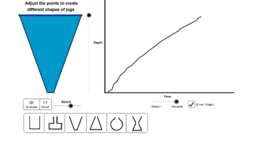Water Pouring Graph

Activity

KS Wong

•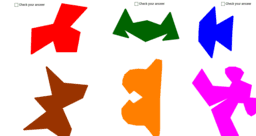Line of Symmetry Activity

Activity

KS Wong

•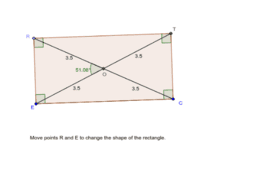Rectangle

Activity

KS Wong

•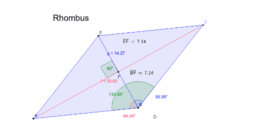Rhombus

Activity

KS Wong

•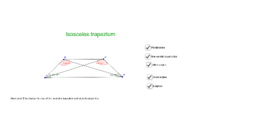Isosceles Trapezium

Activity

KS Wong

•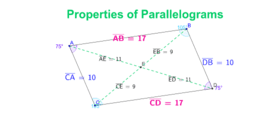Parallelogram

Activity

KS Wong

•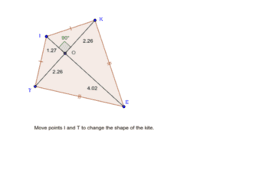Kite

Activity

KS Wong

•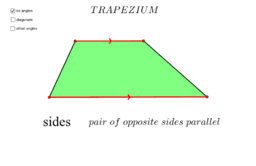Trapezium

Activity

KS Wong

•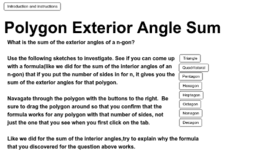Sum of Exterior Angles of A Polygon

Activity

KS Wong

•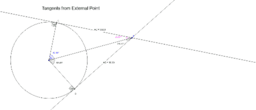Tangents from External Point

Activity

KS Wong

•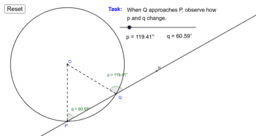Activity

KS Wong

•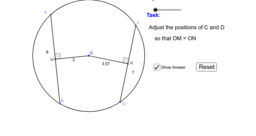Equal Chords, Equidistant from Centre

Activity

KS Wong

•Radius of Circle and Midpoint of Chord

Activity

KS Wong

•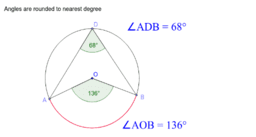Angles Subtended at Centre and Circumference

Activity

KS Wong

•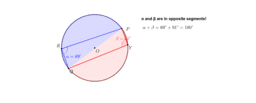Angles in the Same/Opposite segments

Activity

KS Wong

•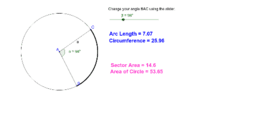Relationship between Angle, Length of Arc & Sector Area

Activity

KS Wong

•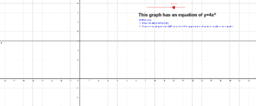Power Function Graphs

Book

KS Wong

•Power Function Graph y=ax^0

Activity

KS Wong

•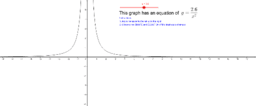Power Function Graph y=a/x^2

Activity

KS Wong

•Power Function Graph y=a/x

Activity

KS Wong

•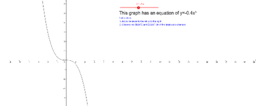Power Function Graph y=ax^3

Activity

KS Wong

•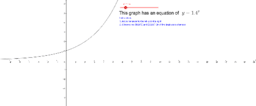Exponential Graphs

Activity

KS Wong

•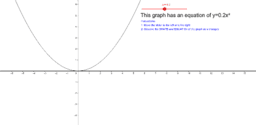Power Function Graph y=ax^2

Activity

KS Wong

•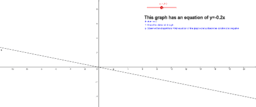Activity

KS Wong